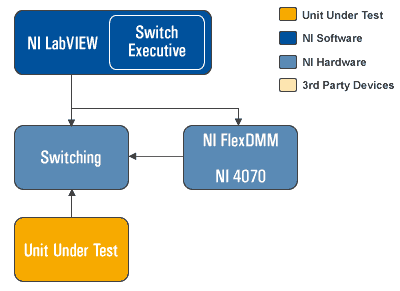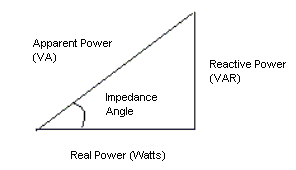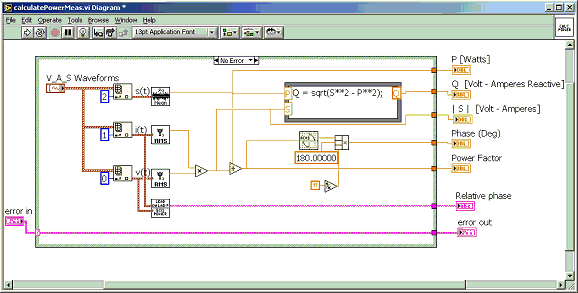# Measure Apparent Power with a Digital Multimeter

Publish Date: Jul 21, 2009 | 12 Ratings | 3.50 out of 5 | Print | 1 Customer Review | Submit your reviewA typical power measurement requires one measurement device to capture the voltage across the terminals of a load, and the second device to capture the current going through the load. However, the actual power calculation depends on the resistive and reactive components (capacitors and/or inductors) in the circuit. The power dissipation in a purely resistive circuit is always a function of the voltage drop and current draw through the circuit.

Reactive circuits appear to function like resistive circuits because they produce voltage drops and draw current. However, reactive circuits actually store or return power. The reactive components cause a phase shift (up to 90 degrees) between the voltage and current waveforms which reduces the overlap between the two curves and effectively delivers less power to the loads. This phenomenon is represented by three different power measurements: reactive power, apparent power, and real power. These three power measurements have a phase relationship that can be visualized in the power triangle, shown below.Figure 1: Power Triangle

Apparent Power
Apparent power is the measure of a circuit’s impedance (Z) and is represented by an S, which has a unit measure of Volt-Amps (VA). Apparent power is the combination of reactive power and real power, without reference to a phase angle. You calculate apparent power by using the following formula:

Apparent Power (S) = Vrms * Irms

To accurately measure apparent power, you need the following capabilities:

• Voltage and current waveform acquisition capability
• Simultaneous acquisition of both measurement waveforms
• Both measurement devices must acquire simultaneously
• Analysis functions

Traditionally, this measurement functionality was only found in specialized power meters because a traditional digital multimeter has asynchronous clocks and does not provide waveform acquisition capability. However, using the modern digital multimeter architecture of the NI PXI-4070 FlexDMM, which boasts digitizer capability, along with NI LabVIEW analysis functions, you can build an apparent power measurement system, as shown in Figure 2. The system requires two PXI-4070 FlexDMMs: one configured to measure voltage and the other to measure current. Using the NI-DMM instrument driver, you can configure two FlexDMMs to trigger off the same PXI trigger line. The block diagram below is an example of how you would make these measurements in LabVIEW.Figure 2: LabVIEW Analysis VIs Used to Calculate Apparent Power

Use The PXI-4070 FlexDMM's 1.8 MS/s Digitizer capability to Make Power Measurements

Customer Reviews
1 Review | Submit your review

good  - Dec 20, 2003

### Ratings

Rate this document